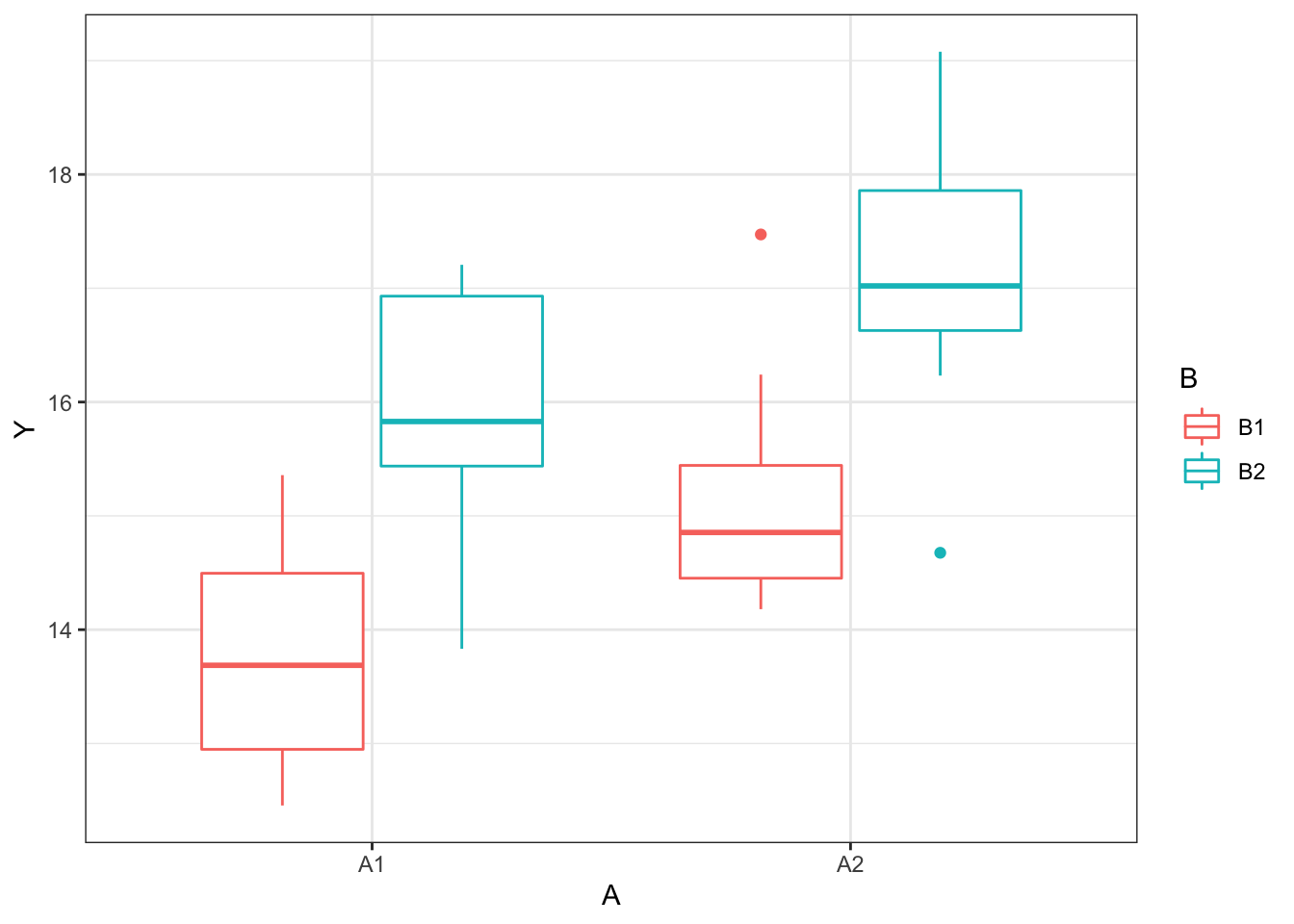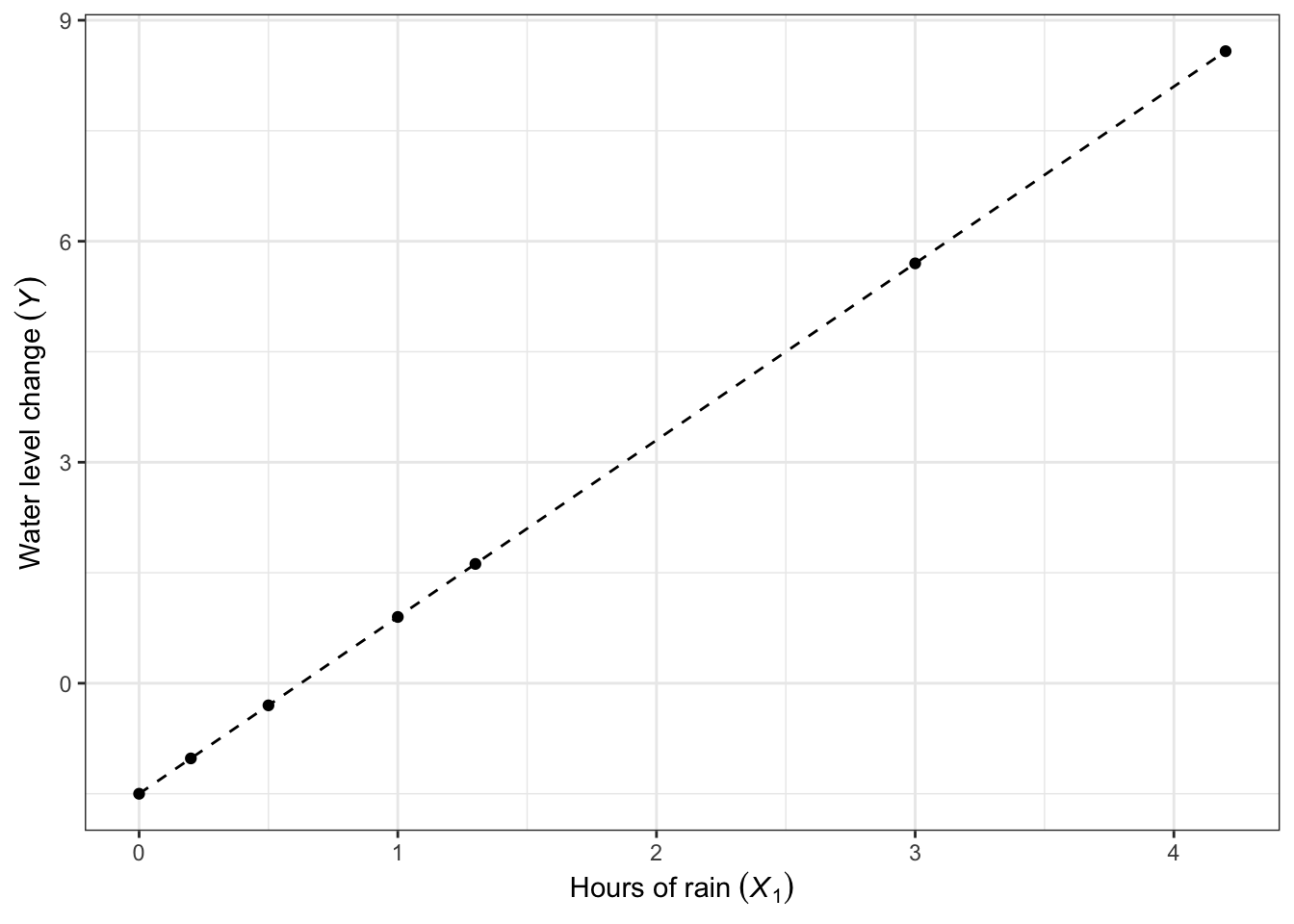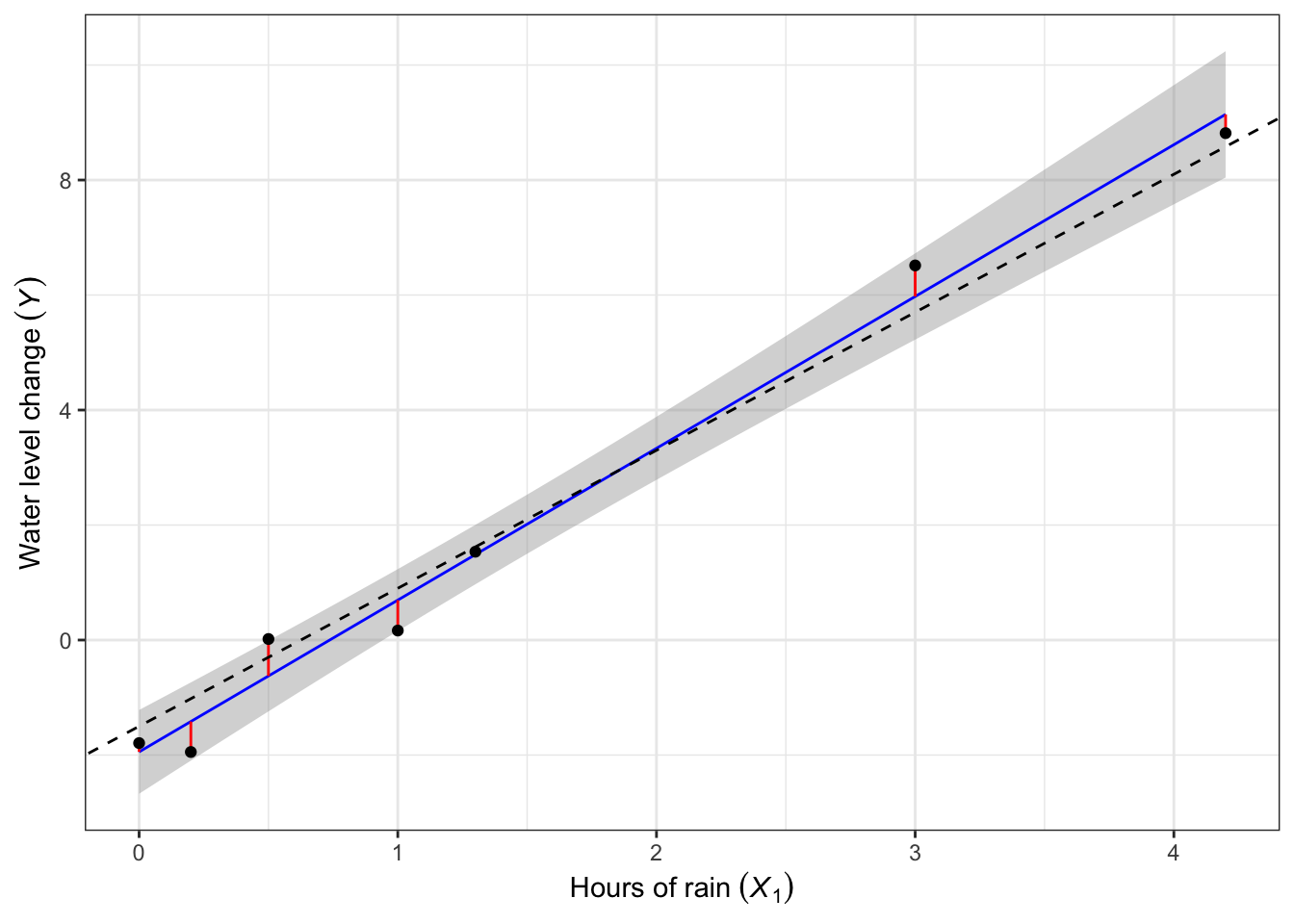## Introduction

The most common tools of experimental data analysis in the behavioral sciences are Analysis of variance (ANOVA), linear regression, or variants thereof.

Both are used to model the effects of a set of independent variables (or predictors) on a continuous dependent variable (such as a score, heart rate, income, response times, ratings, …). Basically, you want to explain and/or predict the DV as a function of the IV(s).

Let us consider an example dataset:The results from an ANOVA may look like this:

summary(aov(Y~A*B, df))
##             Df Sum Sq Mean Sq F value   Pr(>F)
## A            1  15.84   15.84  13.179 0.000873 ***
## B            1  41.53   41.53  34.555 1.01e-06 ***
## A:B          1   0.08    0.08   0.063 0.803633
## Residuals   36  43.27    1.20
## ---
## Signif. codes:  0 '***' 0.001 '**' 0.01 '*' 0.05 '.' 0.1 ' ' 1

The results can be reproduced exactly in a linear regression:

summary(lm(Y~A*B, df))
##
## Call:
## lm(formula = Y ~ A * B, data = df)
##
## Residuals:
##     Min      1Q  Median      3Q     Max
## -2.4411 -0.6553 -0.2642  0.8219  2.3071
##
## Coefficients:
##             Estimate Std. Error t value Pr(>|t|)
## (Intercept) 15.51170    0.17335  89.483  < 2e-16 ***
## A1          -0.62931    0.17335  -3.630 0.000873 ***
## B1          -1.01900    0.17335  -5.878 1.01e-06 ***
## A1:B1       -0.04342    0.17335  -0.250 0.803633
## ---
## Signif. codes:  0 '***' 0.001 '**' 0.01 '*' 0.05 '.' 0.1 ' ' 1
##
## Residual standard error: 1.096 on 36 degrees of freedom
## Multiple R-squared:  0.5704, Adjusted R-squared:  0.5346
## F-statistic: 15.93 on 3 and 36 DF,  p-value: 9.349e-07

This is because the $$F(n,1)$$-distribution is equivalent to the $$t^2_{(n)}$$ distribution. It is only that straightforward for 2-level IVs/factors as there is no direct equivalence for $$F(n,m\neq1)$$ in terms of a $$t$$-distribution.

## Then why use linear regression instead of ANOVA?

In an ANOVA, for each IV you generally get an answer to the question whether any of the levels of that IV vary from the grand mean across all of the levels. You do not know how much or which levels exactly differ (with more than 2 levels). And you usually cannot include continuous IVs (covariates) into the model.

To do these things, you would usually have to conduct post-hoc tests after the ANOVA. Without proper correction, this inflates your statistical error and might appear as “questionable research practices” (QRPs) to some reviewers/peers.

In contrast, linear regression naturally …

• allows covariates (continuous IVs)
• returns effect sizes and p-values in a single model
• tests specific a priori hypotheses
• tells you where the differences are by “deconstructing” the omnibus F-test
• fits a model that may be used to predict outcomes as a function of the IV(s)

## Linear models

Linear models explain the relationship between the DV $$Y$$ and multiple IVs $$X_1,X_2,\ldots,X_n$$, such as $Y=\beta_0 + \beta_1 X_1 + \beta_2 X_2 + \ldots+\beta_n X_n+\epsilon\;,$ where $$\beta_0$$ is the intercept and $$\beta_1,\beta_2,\ldots,\beta_n$$ are the slopes (of $$X_1,X_2,\ldots,X_n$$ on $$Y$$).

The intercept $$\beta_0$$ is equal to all values of $$Y$$ for which $$x_1=x_2=\ldots=x_n=0$$. The residual error is assumed to be normally distributed and zero-centered, $$\epsilon\sim\mathcal{N}(0,\sigma^2)$$.

There is no conceptual difference between so-called simple and multiple linear regressions, they just differ with respect to number of IVs (one vs. more). There are extensions of this framework to account for dependent and/or hierarchical data, such as linear mixed effects. Those are not within the scope of this workshop but the tools discussed here generally apply there as well.

## Linear regression

Linear regression fits the regression weights or coefficients (intercept $$\beta_0$$ and slopes $$\beta_1,\ldots,\beta_n$$) of the linear model to the observation ($$x_1,\ldots,x_n,y$$). It can be used to infer point estimates or confidence intervals for the coefficients as well as p-values for assessing statistical significance.

Model assumptions:

1. Linearity: The relation between $$X$$ and $$Y$$ is linear
2. Independence: Observations (incl. $$Y$$ and $$X$$) are independent, i.e. not sequential, not from the same subject, etc. There are variants such as mixed-effects modeling to handle dependent/hierarchical data
3. Normality: The residuals ($$Y-\hat Y$$, not $$Y$$) should be normally distributed ($$Y-\hat Y \sim \mathcal{N}(0,\sigma)$$)
4. Homoscedasticity: The residual variance is equally distributed across the levels/values of $$X$$

## Linear models: Simple regression example

To water my interior plants, I need approx. 1.5L of water every day from my rainwater tank. I know that during one hour of rain, it rises by 2.4L per hour. Therefore, if we want to predict the daily change of the rainwater tank level $$Y$$ as a function of hours of heavy rain on that day ($$X_1$$), we could expect the following linear relationship:

$Y = -1.5 +2.4 X_1 + \epsilon$

So on a day without any rain ($$x_1=0$$), we expect the change to be -1.5L, as it only decreases by the daily water use ($$\beta_0$$). With each hour of rain, however the water level rises by $$\beta_1$$, so the change on that day may even be positive if more water is deposited than withdrawn.

If we were to record the change in water level $$Y$$ after 0.0, 1.0, 0.5, 3.0, 1.3, 0.2, 4.2 hours of rain ($$X_1$$) on 7 different days, we would expect the following values for $$Y$$:

Day $$x_{1}$$ $$eta_0$$ $$eta_{1}x_{1}$$ $$\epsilon$$ $${Y}$$
1 0.0 -1.5 0.00 0 -1.50
2 1.0 -1.5 2.40 0 0.90
3 0.5 -1.5 1.20 0 -0.30
4 3.0 -1.5 7.20 0 5.70
5 1.3 -1.5 3.12 0 1.62
6 0.2 -1.5 0.48 0 -1.02
7 4.2 -1.5 10.08 0 8.58Knowing the true model, a linear regression should be able to recover the coefficients of the model by fitting it to the simulated observations ($$X_1,Y$$ from table above).

m_e1 <- lm(Y~1+X1, data = example1)
m_e1
##
## Call:
## lm(formula = Y ~ 1 + X1, data = example1)
##
## Coefficients:
## (Intercept)           X1
##        -1.5          2.4

Indeed, the model returns the intercept $$\hat\beta_0=-1.50$$, which is the daily water use and the change of water level on days without rain ($$X_1=0$$), and slope $$\hat\beta_1=2.40$$, which is the increment of water level with each hour of rain on a given day.

## Exercise 1

Head over to maxrabe.com/courses/emsscs. If you have not already done so, follow the instructions to set up an RStudio project for this workshop and install the required packages.

After setting up the RStudio project, download the exercises for day 1 from that same page.

In your R project, open exercise1.r by clicking on the file in the “File” panel on the right (or wherever you moved it). You will find all necessary commands to create the plot and fit the regression to the data.

1. Fit the model by executing all commands in the script. You should recover the “true” values from the beginning of the file.
2. Change the “true values” in line 15 (Y = ...). Does your regression recover those as well?

## Linear models: Residuals

A linear model is rarely a perfect fit to real data. Our simulated observations from the last slide are an extreme exception: We know the “true” composition of $$Y$$ and did not simulate the residual error term $$\epsilon$$. If we do add a normally distributed error on top of the simulations, the observed $$Y$$ will slightly deviate from the predicted $$\hat{Y}$$:

Day $$x_{1}$$ $$eta_0$$ $$eta_{1}x_{1}$$ $$\epsilon$$ $${Y}$$ $$\hat{y}$$
1 0.0 -1.5 0.00 -0.2920852 -1.7920852 -1.9449830
2 1.0 -1.5 2.40 -0.7347356 0.1652644 0.6947592
3 0.5 -1.5 1.20 0.3180176 0.0180176 -0.6251119
4 3.0 -1.5 7.20 0.8146147 6.5146147 5.9742437
5 1.3 -1.5 3.12 -0.0837434 1.5362566 1.4866819
6 0.2 -1.5 0.48 -0.9269293 -1.9469293 -1.4170346
7 4.2 -1.5 10.08 0.2353507 8.8153507 9.1419343In the plot above, we see the observations as black dots and the true model (without error) as the dashed black line. The fitted model is blue. Not all dots are close to the fitted line, due to the residual variance. The difference between the fitted and observed values are visualized as red lines. Due to that residual variance, there is a variety of regression lines within 95% confidence that would be close enough to the observations. This region is visualized as the gray ribbon around the regression line.

##
## Call:
## lm(formula = Y ~ 1 + X1, data = example1a)
##
## Residuals:
##        1        2        3        4        5        6        7
##  0.15290 -0.52949  0.64313  0.54037  0.04957 -0.52989 -0.32658
##
## Coefficients:
##             Estimate Std. Error t value Pr(>|t|)
## (Intercept)  -1.9450     0.2833  -6.866    0.001 **
## X1            2.6397     0.1377  19.169 7.12e-06 ***
## ---
## Signif. codes:  0 '***' 0.001 '**' 0.01 '*' 0.05 '.' 0.1 ' ' 1
##
## Residual standard error: 0.529 on 5 degrees of freedom
## Multiple R-squared:  0.9866, Adjusted R-squared:  0.9839
## F-statistic: 367.4 on 1 and 5 DF,  p-value: 7.124e-06

As we can see, the residual variance has a large impact on the statistical significance of the coefficients. A coefficient is significant if its confidence interval does not contain zero.

We can interpret these results so that both the baseline daily water decrease and the increase by hour of rain are significantly different from zero. If in reality, they were actually zero, an observation this or more extreme simply due to sampling error would be highly unlikely (see p-values).

## Linear models: Categorical differences

The real world is not perfect and we do not know the truth. In reality,

• we have random residuals ($$\epsilon$$) due to measurement error etc.
• we may not really know the baseline daily water use ($$\beta_0$$) or water level increment by hour of rain ($$\beta_1$$), or any true parameter values
• some plants do not need water every day but only on Sundays or so…

We can account for categorical differences in a very similar way! For most days of the week, nothing really changes and the regression model is

$Y = \beta_0 + \beta_1 X_1 + \epsilon\;.$ However, the additional demand on Sundays requires an additional term $$\beta_2$$,

$Y = \beta_0 + \beta_1 X_1 + \beta_2 + \epsilon\;.$ Both regression models can be combined to a single model where $$X_2=1$$ for Sundays and $$X_2=0$$ for Mondays to Saturdays,

$Y = \beta_0 + \beta_1 X_1 + \beta_2 X_2 + \epsilon\;.$

## Linear models: Multiple regression example

Over 21 days, we observed the water level change $$Y$$, hours of rain on that day ($$X_1$$), and whether that day was a Sunday ($$X_2=1$$) or not ($$X_2=0$$):
Day $$x_{1}$$ $$x_{2}$$ $${Y}$$
1 0.23 0 -1.02
2 0.85 0 0.49
3 0.21 0 -0.16
4 0.14 0 -1.09
5 0.68 0 -0.80
6 1.03 0 0.91
7 1.16 1 -0.89
8 0.95 0 0.72
9 0.05 0 -0.98
10 0.37 0 -0.92
11 0.20 0 -0.50
12 0.47 0 -0.20
13 3.26 0 6.12
14 0.18 1 -2.80
15 0.25 0 -1.15
16 1.06 0 0.55
17 0.17 0 -1.69
18 0.16 0 -0.32
19 0.20 0 -1.23
20 0.53 0 0.38
21 0.24 1 -2.85

Plotting the data, we see that the Sunday observations deviate substantially from the regression line: